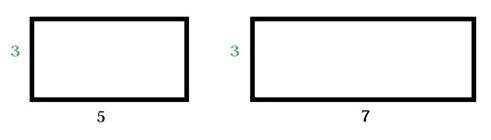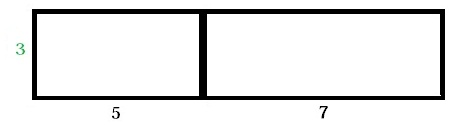# The Distributive property

We begin with an example

Adam has two rectangles with the same height and he wants to know the total area of these two rectangles.He can calculate this in two different ways. Adam can either calculate the areas separately and then add the two areas.

$Rectangle\: 1:\: 3\cdot 5=15$

$Rectangle\: 2:\: 3\cdot 7=21$

$15+21=36$

Or as the height is the same in both rectangles we can multiply the the height with the sum of the bases$3\left ( 5+7 \right )=3\cdot 12=36$

As we can see

$3\cdot 5+3\cdot 7=3\left ( 5+7 \right )=36$

This is an example of the distributive property which can be used to find the product of a number and a sum or a difference.

$a\left ( b+c \right )=\left ( b+c \right )a=ab+ac$

$a\left ( b-c \right )=ab-ac=ba-ca=\left ( b-c \right )a$

The parts of the expression are called terms. A term could either be a number, a variable, or a product. If we have a term that contains both a number and a variable as in 2x the number part of the term, in this case 2, is called the coefficient. A term that only contains a number and no variable part is called a constant term.

If we look at the expression:

$5+3x-2+7x$

This expression has 4 terms where two of the terms are constant terms 5 and -2. The two other terms have the coefficients 3 and 7. Terms like 3x and 7x that have the same variable part are called like terms. The constant terms are like terms as well.

Like terms can be combined as is stated in the distributive property

$3x+7x=\left ( 3+7 \right )x=10x$

Expressions like 3x+7x and 10x are equivalent expressions since they denote the same number.

An expression is written in its simplest form when it contains no like terms and no parentheses

Example

Simplify the expression

$2\left ( 3p+5 \right )-\left ( p+2 \right )$

Notice that the second parenthesis is multiplied by -1. We can instead write the expression as

$2\left ( 3p+5 \right )+\left ( -1 \right )\left ( p+2 \right )$

By using the distributive property we can rewrite the expression as

$6p+10-p-2$

And by combining the like terms we'll get

$5p+8$

Which in this case is the simplest form.

## Video lesson

Simplify the expression

$x (4 - 2) + 3(2x + 1)-(x - 1)$